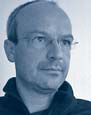# Quantifier Elimination – Statements Can Also Be Calculated

## Quantifier Elimination – Statements Can Also Be Calculated

The understanding of computing on computers has historically evolved in several steps. In the early days of electronic computing equipment, in addition to dealing with large amounts of data, the numerical processing of numbers was in the foreground. The development of computer algebra since the mid 1960s expanded this to computation with symbolic expressions: A simple example is the calculation of (x – 1) . (x + 2) + 2 with the result of x2 + x. That computational work has indeed been performed here, becomes clear, among other things, by the fact that the evaluation of the original expression for a concrete x requires double the amount of arithmetic operations as that of the result. On this basis it became possible, e.g., to automatically derive real functions or even calculate indefinite integrals automatically.

In mathematics, symbolic expressions typically appear in complex statements which can quantify some of the occurring symbols, as for example the following statement about real numbers: For all x there is a y, such that x2 + xy + b > 0 and x + ay2 + b 0. What can it possibly mean to “compute’’ such a statement? Whether our statement is true or not depends on the selection of a and b . We therefore determine the possible choices of a and b for which the statement is true. The result can be rephrased as a statement which no longer contains any kind of quantification: a < 0 and b > 0. Here again, significant computational work has been performed: based on the result, in contrast to the original formulation, one can determine with minimal effort for concrete choices of a and b whether our considered statement is true or not.

Statements about real numbers which contain arithmetic operations, comparisons, logical operations and quantifi cation, as in our example, can always be computed in such a way that the result does not contain any quantification. In the special case where all symbols in a statement are quantified, the result contains no symbols at all; it is then either 0 = 0 (“true’’) or 1 = 0 (“false’’).

Let us consider e.g. a sequence of real numbers as follows: The first two elements x1 and x2 are arbitrary. All others are created according to the rule xn+2 = | xn+1 | – xn. If we start with, say, 1, 2, then we subsequently obtain 1, –1, 0, 1, 1, 0, –1, 1, 2, and we observe that the sequence repeats after the fi rst nine items. This fact can be formulated as a “for all’’ statement about x1, ..., x11. From this, our method computes 0 = 0. This way we automatically prove that for arbitrary starting values the sequence will repeat after the first nine items.

If we consider statements about integral instead of real numbers, then there can be no corresponding software; and this is mathematically provable. In this sense, the methods discussed here border on what is mathematically possible. In addition to integral and real numbers, there are many other domains in the natural sciences which are researched at the Institute in terms of such methods.

This even includes domains in which symbols not only stand for numbers, but also for functions. Corresponding statements can contain arithmetic and derivatives.

This theoretical research is practically implemented in the software system Redlog, which is globally considered as a powerful and efficient computational tool by numerous scientists. The computation time for our above proof about the number sequence is only approximately 0.07 seconds with Redlog.

A recent application of Redlog this year was the analysis of an automatic speed control for cars. Thereby, speed is automatically regulated in such a way that a collision with a preceding vehicle is impossible. Redlog computed starting speeds at which the control can be safely transferred to the system.

Other interesting applications are in the field of the analysis of complex systems in natural sciences, for example electrical networks in physics, reaction systems in chemistry or gene-regulatory networks in biology.

## Thomas SturmRG. 1 Automation of Logic
Phone
+49 681 9325-2920
Email sturm@mpi-inf.mpg.de